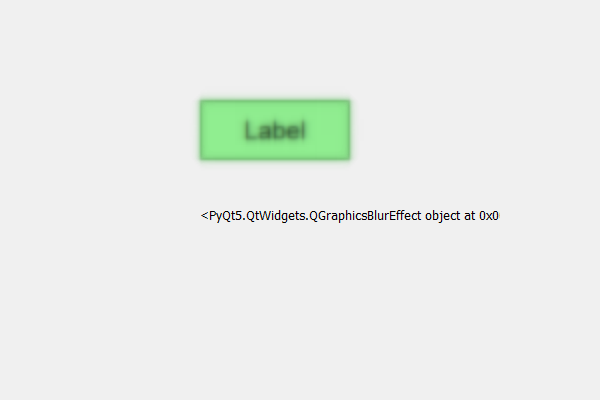# PyQt5 Label – Getting Blur effect object

• Last Updated : 10 May, 2020

In this article we will see how we can get blur effect object of the label by default there is no blur effect to the label although we can create blur effect then add it to the label with the help of `setGraphicsEffect` method.

In order to do this we have to do the following –

Attention geek! Strengthen your foundations with the Python Programming Foundation Course and learn the basics.

To begin with, your interview preparations Enhance your Data Structures concepts with the Python DS Course. And to begin with your Machine Learning Journey, join the Machine Learning - Basic Level Course

1. Create a label
2. Set geometry to the label
3. Create a QGraphicsBlurEffect object
4. Add this object to the label with the help of setGraphicsEffect method
5. Get the opacity object with the help of graphicsEffect method

Note : This object have same properties of the original object

Syntax :

```# creating a blur effect
blur_effect = QGraphicsBlurEffect()

# adding blur effect to the label
label.setGraphicsEffect(blur_effect)

# getting the blur object
object = label.graphicsEffect()
```

Below is the implementation

 `# importing libraries``from` `PyQt5.QtWidgets ``import` `*` `from` `PyQt5 ``import` `QtCore, QtGui``from` `PyQt5.QtGui ``import` `*` `from` `PyQt5.QtCore ``import` `*` `import` `sys`` ` ` ` `class` `Window(QMainWindow):`` ` `    ``def` `__init__(``self``):``        ``super``().__init__()`` ` `        ``# setting title``        ``self``.setWindowTitle(``"Python "``)`` ` `        ``# setting geometry``        ``self``.setGeometry(``100``, ``100``, ``600``, ``400``)`` ` `        ``# calling method``        ``self``.UiComponents()`` ` `        ``# showing all the widgets``        ``self``.show()`` ` `    ``# method for widgets``    ``def` `UiComponents(``self``):`` ` `        ``# creating label``        ``label ``=` `QLabel(``"Label"``, ``self``)`` ` `        ``# setting geometry to the label``        ``label.setGeometry(``200``, ``100``, ``150``, ``60``)`` ` `        ``# setting alignment to the label``        ``label.setAlignment(Qt.AlignCenter)`` ` `        ``# setting font``        ``label.setFont(QFont(``'Arial'``, ``15``))`` ` `        ``# setting style sheet of the label``        ``label.setStyleSheet(``"QLabel"``                            ``"{"``                            ``"border : 2px solid green;"``                            ``"background : lightgreen;"``                            ``"}"``)`` ` `        ``# creating a blur effect``        ``self``.blur_effect ``=` `QGraphicsBlurEffect()`` ` `        ``# adding blur effect to the label``        ``label.setGraphicsEffect(``self``.blur_effect)`` ` `        ``# result label``        ``result ``=` `QLabel(``self``)`` ` `        ``# setting geometry of the result label``        ``result.setGeometry(``200``, ``200``, ``300``, ``30``)`` ` `        ``# getting the blur object``        ``object` `=` `label.graphicsEffect()`` ` `        ``# setting text to the result label``        ``result.setText(``str``(``object``))`` ` ` ` `# create pyqt5 app``App ``=` `QApplication(sys.argv)`` ` `# create the instance of our Window``window ``=` `Window()`` ` `# start the app``sys.exit(App.``exec``())`

Output :My Personal Notes arrow_drop_up